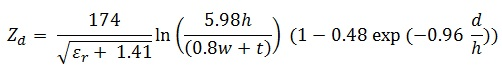## Differential Microstrip Impedance Calculator

 Output of Differential Microstrip Impedance Calculation Z d Ω

The Differential Microstrip Impedance Calculator to measure the opposition to alternating current.

### Differential Microstrip Impedance formulaWhere

d is the trace separation

h is the dielectric thickness

t is the thickness of strip

w is the width of strip

Zd Relative dielectric constant

Er is the dielectric constant of substrate

Thinkcalculator.com provides you helpful and handy calculator resources.